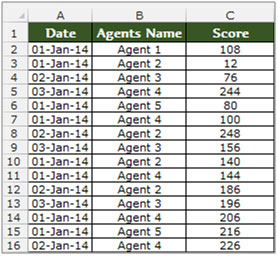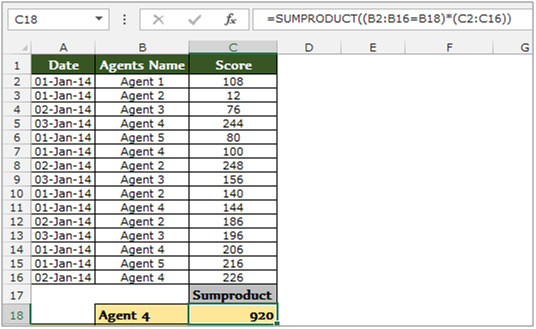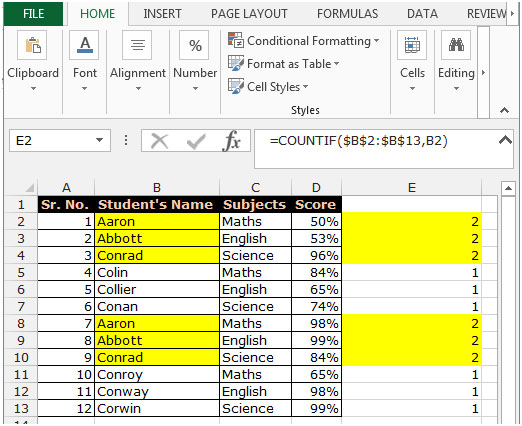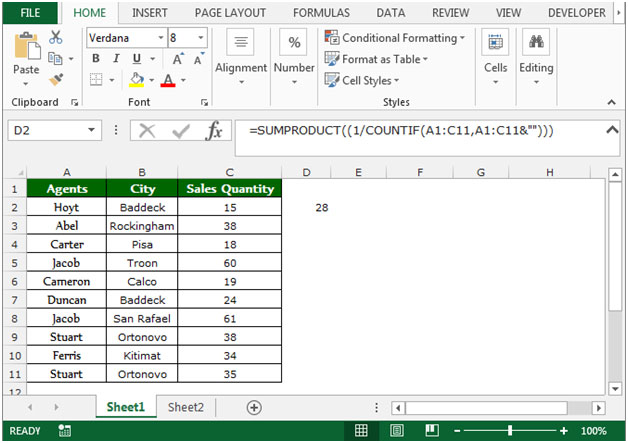# Counting number of unique entries in a specified range in Microsoft Excel

In this article, we will learn how to count the unique entries in a specified range. We use the “SUMPRODUCT” function along with “COUNTIF” function in Microsoft Excel to get the unique values.

SUMPRODUCT: This function is used for adding all the numbers in a range of cells.

The syntax of SUMPRODUCT formula:-  =SUMPRODUCT(array1, [array2],…..)

Let’s understand with a simple exercise how we can use the SUMPRODUCT function with multiple criteria.

We have table in Range “A2 to C16” which contains the Agents’ score data. Column A contains date, Column B Agents’ Name and Column C contains score. Now we need to calculate the total score of Agent 4 in cell C18. Let’s see how we can use the SUMPRODUCT function to get this value.• Select the cell C18, and write the formula in the cell.
• =SUMPRODUCT((B2:B16=B18)*(C2:C16))
• Press Enter on the keyboard.
• The function will return the score value of Agent 4.COUNTIF:- This function is used for counting the duplicity of text or numbers in the range.

The Syntax of COUNTIF:- =COUNTIF(Range,Criteria)

To count the cells which are duplicate in a range, follow below given steps:-

• Select the cell E2 and write the formula.
• =COUNTIF(\$B\$2:\$B\$13,B2)
• Press Enter.
• The function will return 2, which means Aaron is repeating 2 times in Student’s Name column.
• Copy the same formula by pressing the key Ctrl+C and paste in the range E3:E13 by pressing the key Ctrl+V.Let’s take an example to understand how we can count the unique number in a range in Microsoft Excel.

We have data in Range A2:C11 where Column A contains Agent list, column B contains city and column C contains sales quantity.

In this data, if we want to count the number of unique entries in a specified range, then we need to follow below given steps:-

• Select the cell D2.
• Enter the formula in cell =SUMPRODUCT((1/COUNTIF(A1:C11,A1:C11&"")))
• Press Enter on your keyboard.
• The function will return 28, which means 28 entries are unique out of 33 entries.This is all about that how we can count the unique entries & thus avoiding any duplicates in a range in Microsoft Excel 2010 and 2013.

Terms and Conditions of use

The applications/code on this site are distributed as is and without warranties or liability. In no event shall the owner of the copyrights, or the authors of the applications/code be liable for any loss of profit, any problems or any damage resulting from the use or evaluation of the applications/code.